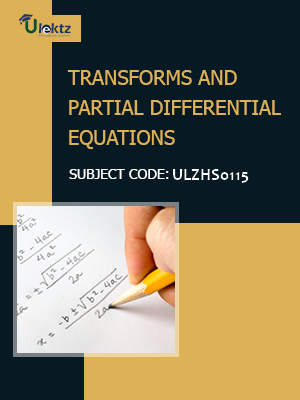•My WalletMy Order
•My Profile
•My Connections
•My Books
•My Videos
•My Tests
•My Calender
•My Messages
•My Shopping Cart
•My Orders
•Account Settings
•Help

# Book Details# Transforms and Partial Differential Equations

 Course Code : ULZHS0115 Author : uLektz University : General for All University Regulation : 2013 Categories : General Engineering Format :ePUB3 (DRM Protected) Type : eBook

FREE

Description :Transforms and Partial Differential Equations of ULZHS0115 covers the latest syllabus prescribed by General for All University for regulation 2013. Author: uLektz, Published by uLektz Learning Solutions Private Limited.

Note : No printed book. Only ebook. Access eBook using uLektz apps for Android, iOS and Windows Desktop PC.

##### Topics
###### UNIT I PARTIAL DIFFERENTIAL EQUATIONS

1.1 Formation of partial differential equations

1.2 Singular integrals

1.3 Solutions of standard types of first order partial differential equations

1.4 Lagrange’s linear equation

1.5 Linear partial differential equations of second and higher order with constant coefficients of both homogeneous and non-homogeneous types.

###### UNIT II FOURIER SERIES

2.1 Dirichlet’s conditions

2.2 General Fourier series

2.3 Odd and even functions

2.4 Half range sine series

2.5 Half range cosine series

2.6 Complex form of Fourier series

2.7 Parseval’s identity

2.8 Harmonic analysis

###### UNIT III APPLICATIONS OF PARTIAL DIFFERENTIAL EQUATIONS

3.1 Classification of PDE

3.2 Method of separation of variables

3.3 Solutions of one dimensional wave equation

3.4 One dimensional equation of heat conduction

3.5 Steady state solution of two dimensional equation of heat conduction

###### UNIT IV FOURIER TRANSFORMS

4.1 Statement of Fourier integral theorem

4.2 Fourier transform pair

4.3 Fourier sine and cosine transforms

4.4 Transforms of simple functions

4.5 Convolution theorem

4.6 Parseval’s identity

###### UNIT V Z - TRANSFORMS AND DIFFERENCE EQUATIONS

5.1 Z-transforms

5.2 Elementary properties

5.3 Inverse Z - transform (using partial fraction and residues)

5.4 Convolution theorem

5.5 Formation of difference equations

5.6 Solution of difference equations using Z - transform.

### Related Books